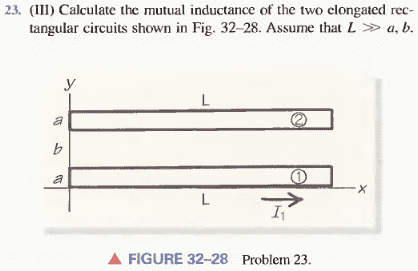# Mutual inductance

Frillth

## Homework StatementΦ = M*I
Biot-Savart law
Ampere's law

## The Attempt at a Solution

I have the formula Φ = M*I, which means that to find the mutual inductance I simply need to find the magnetic flux through the top circuit due to the current in the bottom circuit. However, I don't yet have a solid grasp of Ampere's law. Is there a way to use Ampere's law in this situation, or am I going to have to grind it out with Biot-Savart?

Homework Helper
I have the formula Φ = M*I, which means that to find the mutual inductance I simply need to find the magnetic flux through the top circuit due to the current in the bottom circuit. However, I don't yet have a solid grasp of Ampere's law. Is there a way to use Ampere's law in this situation, or am I going to have to grind it out with Biot-Savart?

Looks like you will have a double integral for each point along the second wire for all the contribution from points on the first, then integrated over its own length.